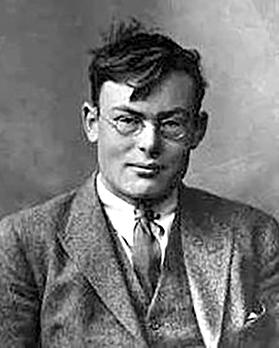Frank P. Ramsey was a British mathematician, philosopher and economist. He was born in Cambridge (UK) in 1903, obtained his Bachelor’s degree in Mathematics from the University of Cambridge (UoC) in 1923, became a mathematics lecturer at UoC in 1926 and died in 1930 at the age of 26 after developing jaundice.Image source: https://en.wikipedia.org/wiki/Frank_P._Ramsey

The Ramsey number R(r,s) is the smallest number n such that, for every set of n objects with every two being related or unrelated, there is an r-clique (r objects, every two of which are related) or an s-coclique (s objects, no two of which are related). Motivated by a problem in formal logic, Ramsey showed that R(r,s) exists for any r and s (F. P. Ramsey, On a problem of formal logic, Proceedings of the London Mathematical Society 30 (1930), 264-286). This gave rise to Ramsey Theory, a popular branch of combinatorial mathematics described as finding order in disorder. A central problem is to determine R(r,s) for any given r and s. R(r,s) is known for a small number of cases.